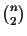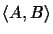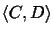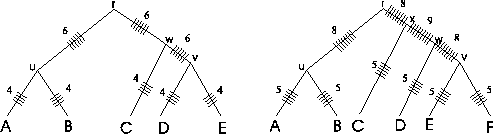Next: Methods Up: Introduction Previous: Scoring Pairwise Sequence Alignments

## Sum-of-Pairs Measure

To calculate the score with the SP measure [Carillo and Lipman, 1988], allscores of the pairwise alignments within the MSA (see Definition 2.6) are added up. SP methods are obviously deficient from an evolutionary perspective [Altschul and Lipman, 1989]. Consider a tree (Figure 2) constructed for a family containing five proteins. The score of a pairwise alignmentevaluates the probability of evolutionary events on edges (u, A) and (u, B) of the tree; that is, the edges that represent the evolutionary distance between sequence A and sequence B. Likewise, the score of a pairwise alignmentevaluates the likelihood of evolutionary events on edges (C, w), (w, v) and (v, D) of the tree.By adding "ticks" to the evolutionary tree that are drawn each time an edge is evaluated when calculating the SP score (Figure 2), it is readily seen that with the SP method different edges of the evolutionary tree of the protein family are counted a different numbers of times. In the example tree on the left side that corresponds to the MSA of figure 1, edges (r, u), (r, w) and (w, v) are each counted six times by the SP method, while edges (u, A), (u, B), (v, D), (v, E), and (w, C) are each counted four times. The numbers on the edges are the numbers of "ticks". It gets worse as the tree grows (see tree on the right). There is no theoretical justification to suggest that some evolutionary events are more important than other ones. Thus, SP methods are intrinsically problematic from an evolutionarily perspective for scoring MSAs. This was the motivation to developed a scoring method that evaluates each edge equally. In addition, we wanted a scoring function that does not depend on the actual tree structure. We report here a circular sum'' (or CS) method for evaluating the quality of an MSA. The method uses a solution to the Traveling Salesman Problem, which identifies a circular tour through an evolutionary tree connecting the sequences in a protein family. The algorithm gives an upper bound, the best score that can possibly be achieved by any MSA for a given set of protein sequences. Both the bound and the circular tour can be derived without an explicit knowledge of the correct evolutionary tree; thus the method can be applied without need to address the mathematical issues involved in tree construction. Last, it gives us an absolute score of MSAs which is important in designing and verifying MSA heuristics. Both the tree construction problem and the Traveling Salesman Problem are NP complete. But the Traveling Salesman Problem (see next section) has been studied very extensively [Johnson, 1990,Johnson, 1987], and optimal solutions can be calculated within a few hours for up to 1000 cities and in a few seconds for up to 100 cities, whereas the construction of evolutionary trees is still a big problem. There are heuristics for large scale problems that calculate near optimal solutions that are within 1% to 2% of the optimum [Padberg and Rinaldi, 1991,Groetschel and Holland, 1991].Next: Methods Up: Introduction Previous: Scoring Pairwise Sequence Alignments
Chantal Korostensky
1999-07-14